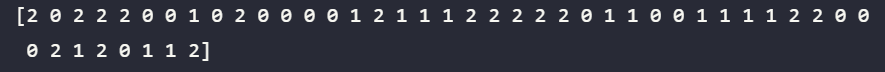# Implementing KNN with Scikit Learn for Beginners

Here you will find how to implement a simple Classification(Supervised Learning) algorithm called KNN, which stands for K-Nearest Neighbour.

We will be using the sci-kit-learn library in python. It’s one of the most widely used ML libraries in python.

# What is KNN?

KNN stands for K-Nearest Neighbors. This is Supervised learning, Classification Algorithm. Let me briefly elaborate on what these terms mean.

Supervised learning is a part of Machine learning where we train our model with data that has the target variable or the variable of our interest in it. For example, for Implementing KNN in this article, I am going to use Iris data.w Which has five columns with the first four columns representing the flower Iris’s length and width of its sepal and petal. The fifth column represents which species of iris does the sample belongs to. This column is our target variable or the variable which our ML model should predict.

Since Iris data also has a target variable in it we have to use Supervised learning techniques. Depending on the type of target variable we have to use either Regression or Classification algorithms.

• If the target variable is Continuous we will use Regression.
• If the target variable is Discrete we will use Classification.

K Nearest Neighbour is a simple algorithm that stores all the available cases and classifies the new data or case based on a similarity measure. It is mostly used to classify a data point based on how its neighbors are classified. And the K in KNN stands for the number of neighbors that we choose to compare our sample with.

SciKit-learn library has some datasets preloaded in it. And Iris's data set is one of those. The following blocks of code deal with loading the Iris data set.

I highly recommend installing Anaconda Distribution in your system instead of plain Python 3. Now let's get on with code.

Now, let's put the data into two Data Frames. One coating the features and the other containing the target variable.

# Splitting the data into training and testing data.

The idea behind splitting the data into two sets that is training and testing comes from logic, that the true measure of our model’s performance can only be gauged with data which is not been seen by our trained model.

So, we split the data into training data and testing data to train and test our model separately. We can do these using pandas, but the sklearn library has a much better option in the form of train_test_split.

# Fitting(Training) & Predicting using SKlearn models.

## General Overview:

Almost all the Machine learning models also called estimators in the SKlearn module have three basic steps. With each model having its own quirks and parameters.

STEP 1: Call the model

STEP 2: Train the model also called the fitting model and this can be done using .fit() method.

STEP 3: Predicting target value using the trained model. This can be done using .predict() method.

## Training the model & Predicting target variable.Predicted Values. 0,1,2 to stands for Iris species of Setosa, Virginica, Versicolor respectively.

## A small note on Hyperparameter tuning:

The optimal value of n_neighbors or K value is something that should be decided by the Data Scientist. Different ML models have similar types of parameters that we need to tune to get an optimal model. These parameters are called Hyperparameters and tuning of these parameters is called Hyperparameter Tuning.

There are several methods to do this but this is not the scope of this article. But I plan to write an article on this is near future.

# Accuracy of the Model.

Now how do we check the performance of the model? For this, we need some metrics to quantify the performance of the model. One such metric is Accuracy.

Accuracy is the ratio of the number of correctly predicted target values to the number of predictions done by the model. We can calculate the accuracy of the model using .score method.

An accuracy of 97% is not bad for first out of box model right!!

# Conclusion:

With this, we have completed a simple cycle in Machine Learning. You can get all the code in this Git Repo. Thank you for reading! I hope you have gained something from this article. Please do clap and follow me. You can also find these articles on sivachandan.com

--

--